Courses

# Test: Communication Systems - 3

## 25 Questions MCQ Test Communication Theory | Test: Communication Systems - 3

Description
This mock test of Test: Communication Systems - 3 for Electronics and Communication Engineering (ECE) helps you for every Electronics and Communication Engineering (ECE) entrance exam. This contains 25 Multiple Choice Questions for Electronics and Communication Engineering (ECE) Test: Communication Systems - 3 (mcq) to study with solutions a complete question bank. The solved questions answers in this Test: Communication Systems - 3 quiz give you a good mix of easy questions and tough questions. Electronics and Communication Engineering (ECE) students definitely take this Test: Communication Systems - 3 exercise for a better result in the exam. You can find other Test: Communication Systems - 3 extra questions, long questions & short questions for Electronics and Communication Engineering (ECE) on EduRev as well by searching above.
QUESTION: 1

### The output resistance of a microphone is 20 ohms. It is to feed a line of impedance 320 ohms. To ensure maximum power transfer, the turn ratio of transformer is

Solution:

(20)(4)2 = 320 ohms.

QUESTION: 2

### In a reactance FET, gm = 9 x 10-3 Siemens, gate to source resistance is one-ninth of reactance of gate to drain capacitor and frequency is 4 MHz. The capacitive-reactance is

Solution: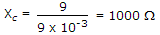.

QUESTION: 3

### Audio cassettes used in household tape recorders have a width of

Solution:

Home tape recorders use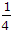in (i.e., 6.3 mm) tape having two tracks of 2.5 mm width each with an in between separation of 1.3 mm.

QUESTION: 4

The receiver of a pulsed RADAR can detect signal power in the order of

Solution:

It can detect very low power signals.

QUESTION: 5

In a 100% amplitude modulated signal, the power in the upper sideband when carrier power is to be 100 W and modulation system SSBSC, is

Solution:

Modulation index = 100%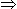1

Pc = 100 W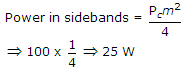QUESTION: 6

Which camera tube has highest dark current?

Solution:

Dark current is the small signal current even when there is no illumination on the face plate of camera tube.

QUESTION: 7

If a sound wave has a frequency of 50 Hz, the wavelength is

Solution: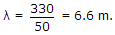QUESTION: 8

Skin depth at 1000 MHz in comparison with that at 500 MHz is

Solution:

Skin depth decreases as square root increase in frequency.

QUESTION: 9

Consider the following

1. Generation of SSB signals
2. Design of minimum phase type filters
3. Representation of band pass signals
Which of the above applications is Hilbert transform used?
Solution:

Hilbert transform is used in all the applications.

QUESTION: 10

The dc voltage on plate modulated class C amplifier is 8 kV. If the peak modulating voltage is 4 kV, the peak RF voltage delivered to load is

Solution:

Peak RF voltage delivered to load = 2 (8 + 4) = 24 kV.

QUESTION: 11

In telephone systems the relation between load r, number of calls initiated during a specified interval λ and average duration of a call (h) is

Solution:

Load = (number of calls) (duration of call).

QUESTION: 12

In a Vidicon camera tube

Solution:

Grid G2 accelerates the electrons. Grid G3, is focus grid and slows the electrons so that they fall perpendicularly on the target at a low speed without causing secondary emission.

QUESTION: 13

In a CD the sampling frequency is nearly

Solution:

For proper sampling and faithful reproduction sampling frequency must be at least twice the highest frequency in the signal.

QUESTION: 14

Which of the following is present in both TRF receiver and superheterodyne receiver?

Solution:

Detection (also called demodulation) is an essential function.

QUESTION: 15

Consider the following statements about loop antenna

1. It is suitable for direction finding application
2. It is a single turn or multiturn coil carrying RF current
3. The loop is surrounded by a magnetic field everywhere perpendicular to the loop
Which of the above statements are correct?
Solution:

All the three are correct.

QUESTION: 16

The number of channels in a stereophonic system is

Solution:

Left and right channels.

QUESTION: 17

When the carrier is unmodulated, a certain transmitter radiates 9 kW when the carrier is sinusoidally modulated the transmitter radiates 10.125 kW. The modulation index will be

Solution: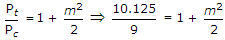.

QUESTION: 18

A signal x(t) = 100 cos (24p x 103t) is ideally sampled with a sampling period of 50 μ sec and then passed through an ideal low pass filter with cut off frequency of 15 kHz Which of the following frequencies are present at the filter output?

Solution: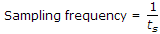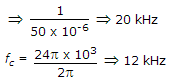Frequency pass by L.P.F. are, fc fs - fc12 kHz, 8 kHz.

QUESTION: 19

A sinusoidal voltage of 2 kV peak value is amplitude modulated to give 20% modulation. The peak value of each side band term is

Solution: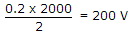.

QUESTION: 20

Consider the following reasons for using antenna coupler

1. To prevent illegal radiation of spurious frequencies
2. To tune out reactive components of antenna impedance
3. To have correct value of load impedance for transmitter.
Which of the above are correct?
Solution:

All the three are correct.

QUESTION: 21

In a high speed modem the data rate handled is about

Solution:

When data rate is in the range of 2400 to about 10800 bits per second, modem is designated as high speed.

QUESTION: 22

Which of the following is true in a tape recorder?

Solution:

Small variation (less than 6%) in motor speed causes vow and large variation (more than 6%) in motor speed causes flutter.

QUESTION: 23

Trinitron is a monochrome picture tube.

Solution:

Frequency band for local programs is fixed.

QUESTION: 24

The first pulse in a TV remote control is

Solution:

The first pulse is preliminary pulse followed by start pulse.

QUESTION: 25

If the ratio of ac to dc load impedance of a diode detector is low, the result will be

Solution:

Negative peak will be clipped.